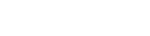# Godot Tips and Tricks

Learn new tips and tricks when using Godot.

## GDScript Tips and Tricks

When you want to repeat a loop `NUM_TIMES` times, simply use this code rather than range.

``````const NUM_TIMES = 8

for n in NUM_TIMES:
# Do stuff
print(n)
``````

Print debug text for multiple items with `prints("Items:", item1, item2, item3)`. This separates the items with spaces.

Convert a number to a string with `str(number)`.

Return multiple data values from a function by returning a dictionary or array.

``````func add_one(a, b):
return [a + 1, b + 1]

return { "a": a + 2, "b": b + 2 }
``````

Check for an odd or even integer value.

``````func is_even(x: int):
return x % 2 == 0

func is_odd(x: int):
return x % 2 != 0
``````

Get a coordinate of a bounding grid square of a point, and offset into the grid square.

``````func box_x(x: int):
return x / BOX_WIDTH * BOX_WIDTH # Use integer division

func x_offset(x: int):
return x % BOX_WIDTH
``````

Use infinite values.

``````func divide_x_by_y(x, y):
if x == 0:
return x
if y == 0:
return INF
return x / y

print(-10e8 > -INF)
``````

Use assertions to halt debug code if there is an unexpected value.

``````assert(x > 6) # Breaks and outputs an error if x <= 6
``````

Adopt a meaningful naming convention for constants, variables, and functions such as: `MAX_SIZE, x_offset, generate_enemy()`.

Avoid prefixing with `get_` and `set_` since this makes it hard to get your function names to show up in auto-suggest.

Consider adding a trailing underscore char `_` to local function var names to ensure that they don’t shadow external var names, and you don’t need to expend much effort to name your vars.

Avoid overuse of static typing. Code may be more elegant when you use variants, and you may thus change the type without creating a new variable with a new name.